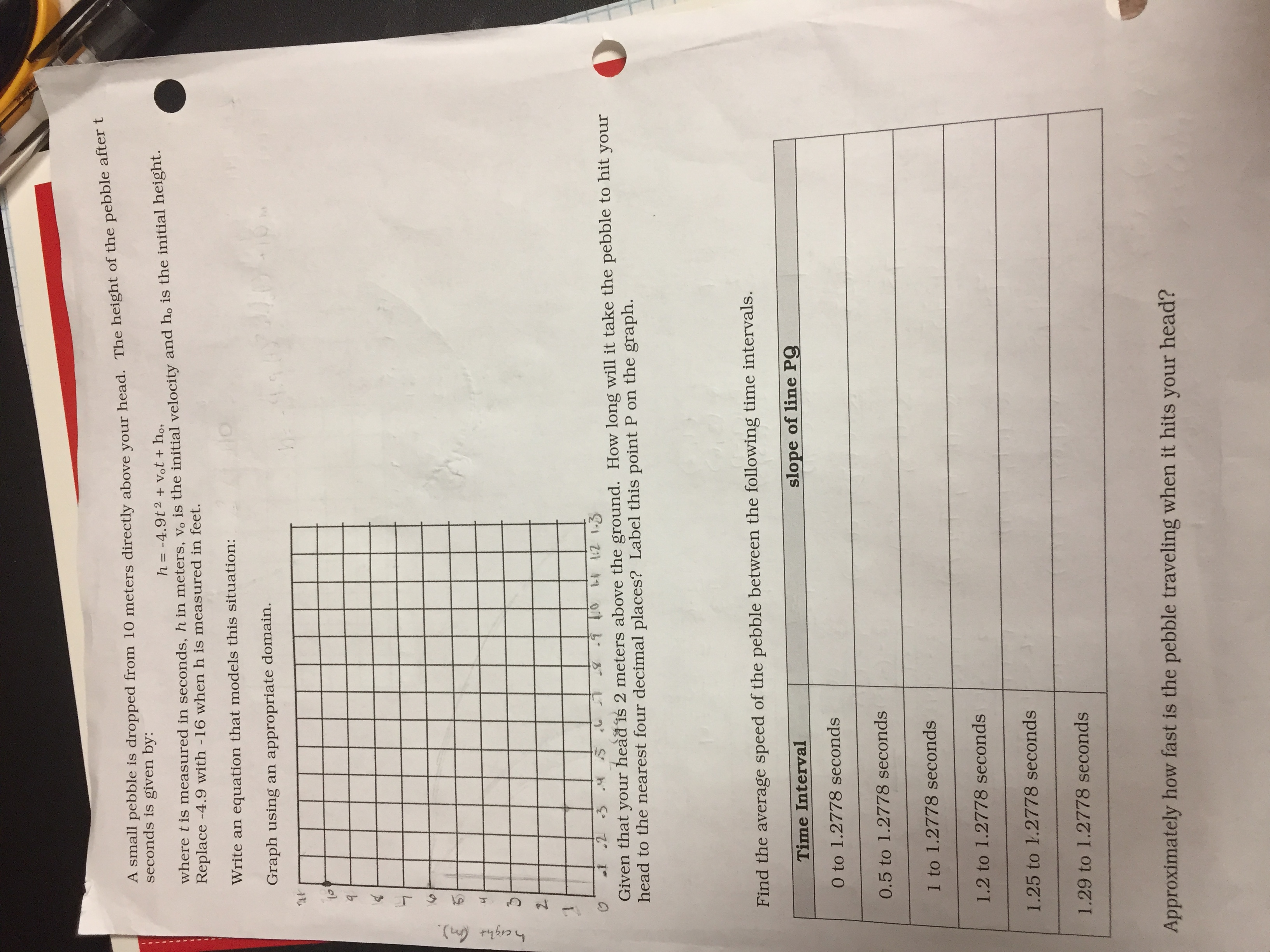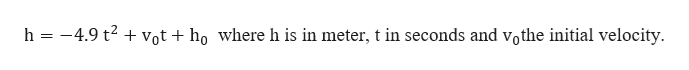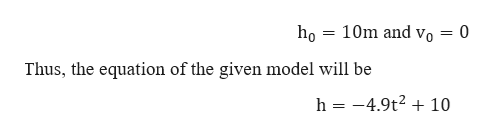# A small pebble is dropped from 10 meters directly above your head. The height of the pebble after tseconds is given by:h= -4.9t2 + Vot+ ho,where t is measured in seconds, h in meters, Vo is the initial velocity and ho is the initial height.Replace -4.9 with -16 when h is measured in feet.Write anequation that models this situation:Graph using an appropriate domain.2I-12 1.3.1 .2 3Given that your head is 2 meters above the ground. How long will it take the pebble to hit yourhead to the nearest four decimal places? Label this point P on the graph.Find the average speed of the pebble between the following time intervals.slope of line PgTime Interval0 to 1.2778 seconds0.5 to 1.2778 seconds1 to 1.2778 seconds1.2 to 1.2778 seconds1.25 to 1.2778 seconds1.29 to 1.2778 secondsApproximately how fast is the pebble traveling when it hits your head?A

Question
9 views

My question is: what equation will model a situation where a pebble is dropped from 10 meters directly above someone's head when the height of the pebble after "t" seconds is given by h=-4.9t^2+vt+h? Here is a picture of my assignment...help_outlineImage TranscriptioncloseA small pebble is dropped from 10 meters directly above your head. The height of the pebble after t seconds is given by: h= -4.9t2 + Vot+ ho, where t is measured in seconds, h in meters, Vo is the initial velocity and ho is the initial height. Replace -4.9 with -16 when h is measured in feet. Write an equation that models this situation: Graph using an appropriate domain. 2 I -12 1.3 .1 .2 3 Given that your head is 2 meters above the ground. How long will it take the pebble to hit your head to the nearest four decimal places? Label this point P on the graph. Find the average speed of the pebble between the following time intervals. slope of line Pg Time Interval 0 to 1.2778 seconds 0.5 to 1.2778 seconds 1 to 1.2778 seconds 1.2 to 1.2778 seconds 1.25 to 1.2778 seconds 1.29 to 1.2778 seconds Approximately how fast is the pebble traveling when it hits your head? A fullscreen
check_circle

star
star
star
star
star
1 Rating
Step 1

Given the height of pebble after t seconds is given byhelp_outlineImage Transcriptioncloseh -4.9 t2Vot + họ where h is in meter, t in seconds and vothe initial velocity. fullscreen
Step 2

Now, initially the pebble is at rest, so the height and the velocity will behelp_outlineImage Transcriptionclose10m and vo 0 Thus, the equation of the given model will be h -4.9t2 10 fullscreen
Step 3

Now we plot the equation, with time a...

### Want to see the full answer?

See Solution

#### Want to see this answer and more?

Solutions are written by subject experts who are available 24/7. Questions are typically answered within 1 hour.*

See Solution
*Response times may vary by subject and question.
Tagged in

### Derivative